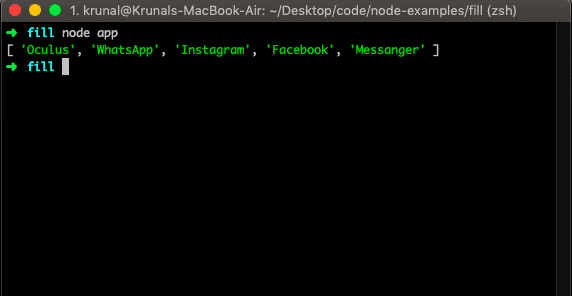# JavaScript unshift: The Complete Guide

1
47JavaScript unshift() method is like the push() method, only it works at the beginning of the array. The unshift() method can prepend one or more elements to the beginning of an array. This alters an array on which the method was called.

## Javascript unshift

The array unshift() is a built-in JavaScript function that adds elements to the beginning of the array and returns the new length of the array. The unshift() method takes several arguments, the same as the number of elements to be added.

The unshift() method changes the array’s length directly, so it is not a pure function.

If we need to add a new item at the end of the array, we can use the array push() method.

When people first start to learn about arrays in javascript then, they usually learn about the JavaScript push() and JavaScript pop() methods which merely add and remove the item from the end of an array.

Suppose their particular language supports it. Then, these two methods append the items to the array and remove an element from the array, respectively.

These methods work at the end of the array and not at the start of an array, where the index is largest.

Javascript arrays have the native support for this push() and pop(), two methods. Javascript also supports parallel methods that work at the beginning of the array, where the index is the smallest.

Javascript unshift and JavaScript shift() methods are the same as push() and pop(), only work at the other end of the array.

### Syntax

The syntax of the array.unshift() method is the following.

`array.unshift(item1, item2, ..., itemX)`

### Parameters

The number of arguments to this function is the same as the number of elements added at the beginning of the array.

### Return value

This function returns the new length of the array after inserting the arguments at the beginning of the array.

### Example

Let us take a simple example and see how it works. Write the following code in the app.js file. We are using node.js to test the Javascript code.

```// app.js

let apps = ["Instagram", "Facebook", "Messanger"];
apps.unshift("Oculus", "WhatsApp");

console.log(apps);```

Run the file, and you can see that Oculus and WhatsApp are appended at the start of an array.The following code will include almost all the scenarios of the unshift() method.

```let arr = [1, 2];

arr.unshift(0); // result of call is 3, the new array length
// arr is [0, 1, 2]

arr.unshift(-2, -1); // = 5
// arr is [-2, -1, 0, 1, 2]

arr.unshift([-3]);
// arr is [[-3], -2, -1, 0, 1, 2]```

## Javascript array unshift object.

Let’s take an example in which we defined two objects and added them into one array. Then try to add a third object using an array unshift() method.

```// app.js

const objA = {
name: 'Millie Bobby Brown'
}
const objB = {
name: 'Finn Wolfhard'
}
const objC = {
}
let arr = [objA, objB];
arr.unshift(objC);
console.log(arr);
```

See the output.

```➜  es git:(master) ✗ node app
[ { name: 'Sadie Sink' },
{ name: 'Millie Bobby Brown' },
{ name: 'Finn Wolfhard' } ]
➜  es git:(master) ✗
```

## Unshift multiple array items in Javascript

We can add multiple items to the start of an array using the unshift() method.

```// app.js

const objA = {
name: 'Millie Bobby Brown'
}
const objB = {
name: 'Finn Wolfhard'
}
const objC = {
}
const objD = {
name: 'Noah Schnapp'
}
const objE = {
name: 'Gaten Matterazo'
}
let arr = [objA, objB];
arr.unshift(objC, objD, objE);
console.log(arr);
```

See the output.

```➜  es git:(master) ✗ node app
[ { name: 'Sadie Sink' },
{ name: 'Noah Schnapp' },
{ name: 'Gaten Matterazo' },
{ name: 'Millie Bobby Brown' },
{ name: 'Finn Wolfhard' } ]
➜  es git:(master) ✗
```

## Conclusion

The array unshift() method is used to prepend the item or items into the array.

That is it for the unshift() method tutorial.

## Recommended Posts

JavaScript array concat()

JavaScript array every()

JavaScript array reverse()

JavaScript array reduce()

JavaScript array includes()

#### 1 Comment

1.Amit Parmar

thanks for the guide

This site uses Akismet to reduce spam. Learn how your comment data is processed.